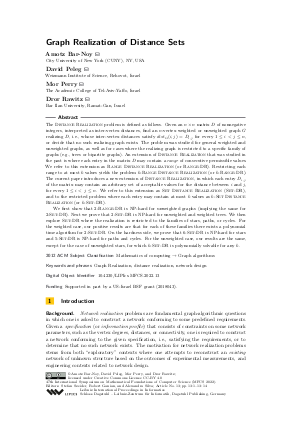Document# Graph Realization of Distance Sets

### Authors Amotz Bar-Noy, David Peleg, Mor Perry, Dror Rawitz## File

LIPIcs.MFCS.2022.13.pdf
• Filesize: 0.63 MB
• 14 pages

## Cite As

Amotz Bar-Noy, David Peleg, Mor Perry, and Dror Rawitz. Graph Realization of Distance Sets. In 47th International Symposium on Mathematical Foundations of Computer Science (MFCS 2022). Leibniz International Proceedings in Informatics (LIPIcs), Volume 241, pp. 13:1-13:14, Schloss Dagstuhl - Leibniz-Zentrum für Informatik (2022)
https://doi.org/10.4230/LIPIcs.MFCS.2022.13

## Abstract

The Distance Realization problem is defined as follows. Given an n × n matrix D of nonnegative integers, interpreted as inter-vertex distances, find an n-vertex weighted or unweighted graph G realizing D, i.e., whose inter-vertex distances satisfy dist_G(i,j) = D_{i,j} for every 1 ≤ i < j ≤ n, or decide that no such realizing graph exists. The problem was studied for general weighted and unweighted graphs, as well as for cases where the realizing graph is restricted to a specific family of graphs (e.g., trees or bipartite graphs). An extension of Distance Realization that was studied in the past is where each entry in the matrix D may contain a range of consecutive permissible values. We refer to this extension as Range Distance Realization (or Range-DR). Restricting each range to at most k values yields the problem k-Range Distance Realization (or k-Range-DR). The current paper introduces a new extension of Distance Realization, in which each entry D_{i,j} of the matrix may contain an arbitrary set of acceptable values for the distance between i and j, for every 1 ≤ i < j ≤ n. We refer to this extension as Set Distance Realization (Set-DR), and to the restricted problem where each entry may contain at most k values as k-Set Distance Realization (or k-Set-DR). We first show that 2-Range-DR is NP-hard for unweighted graphs (implying the same for 2-Set-DR). Next we prove that 2-Set-DR is NP-hard for unweighted and weighted trees. We then explore Set-DR where the realization is restricted to the families of stars, paths, or cycles. For the weighted case, our positive results are that for each of these families there exists a polynomial time algorithm for 2-Set-DR. On the hardness side, we prove that 6-Set-DR is NP-hard for stars and 5-Set-DR is NP-hard for paths and cycles. For the unweighted case, our results are the same, except for the case of unweighted stars, for which k-Set-DR is polynomially solvable for any k.

## Subject Classification

##### ACM Subject Classification
• Mathematics of computing → Graph algorithms
##### Keywords
• Graph Realization
• distance realization
• network design

## Metrics

• Access Statistics
• Total Accesses (updated on a weekly basis)
0

## References

1. Ingo Althöfer. On optimal realizations of finite metric spaces by graphs. Discret. Comput. Geom., 3:103-122, 1988.2. Agnese Baldisserri. Buneman’s theorem for trees with exactly n vertices. CoRR, 2014. URL: http://arxiv.org/abs/1407.0048.
3. Hans-Jürgen Bandelt. Recognition of tree metrics. SIAM J. Discret. Math., 3(1):1-6, 1990.4. Amotz Bar-Noy, David Peleg, Mor Perry, and Dror Rawitz. Composed degree-distance realizations of graphs. In 32nd IWOCA, volume 12757 of LNCS, pages 63-77. Springer, 2021.5. Reuven Bar-Yehuda and Dror Rawitz. Efficient algorithms for integer programs with two variables per constraint. Algorithmica, 29(4):595-609, 2001.6. Fan R. K. Chung, Mark W. Garrett, Ronald L. Graham, and David Shallcross. Distance realization problems with applications to internet tomography. J. Comput. Syst. Sci., 63(3):432-448, 2001. URL: https://doi.org/10.1006/jcss.2001.1785.
7. Joseph C. Culberson and Piotr Rudnicki. A fast algorithm for constructing trees from distance matrices. Inf. Process. Lett., 30(4):215-220, 1989.8. Elias Dahlhaus. Fast parallel recognition of ultrametrics and tree metrics. SIAM J. Discret. Math., 6(4):523-532, 1993.9. Andreas W. M. Dress. Trees, tight extensions of metric spaces, and the cohomological dimension of certain groups: a note on combinatorial properties of metric spaces. Adv. in Math., 53:321-402, 1984.10. Shimon Even, Alon Itai, and Adi Shamir. On the complexity of timetable and multicommodity flow problems. SIAM J. Comput., 5(4):691-703, 1976.11. Tomás Feder, Adam Meyerson, Rajeev Motwani, Liadan O'Callaghan, and Rina Panigrahy. Representing graph metrics with fewest edges. In 20th STACS, pages 355-366. Springer, 2003.12. S. Louis Hakimi and S. S. Yau. Distance matrix of a graph and its realizability. Quart. Appl. Math., 22:305-317, 1965.13. Juhani Nieminen. Realizing the distance matrix of a graph. J. Inf. Process. Cybern., 12(1/2):29-31, 1976.14. A. N. Patrinos and S. L. Hakimi. The distance matrix of a graph nand its tree realizability. Quarterly of Applied Math., 255, 1972.15. Elena Rubei. Weighted graphs with distances in given ranges. J. Classif., 33:282-297, 2016.16. J. M. S. Simões-Pereira. An optimality criterion for graph embeddings of metrics. SIAM J. Discret. Math., 1(2):223-229, 1988.17. J. M. S. Simões-Pereira. An algorithm and its role in the study of optimal graph realizations of distance matrices. Discret. Math., 79(3):299-312, 1990.18. Hiroshi Tamura, Masakazu Sengoku, Shoji Shinoda, and Takeo Abe. Realization of a network from the upper and lower bounds of the distances (or capacities) between vertices. In Proc. IEEE Int. Symp. on Circuits and Systems (ISCAS), pages 2545-2548. IEEE, 1993.19. Sacha C. Varone. A constructive algorithm for realizing a distance matrix. Eur. J. Oper. Res., 174:102-111, 2006.X

Feedback for Dagstuhl Publishing